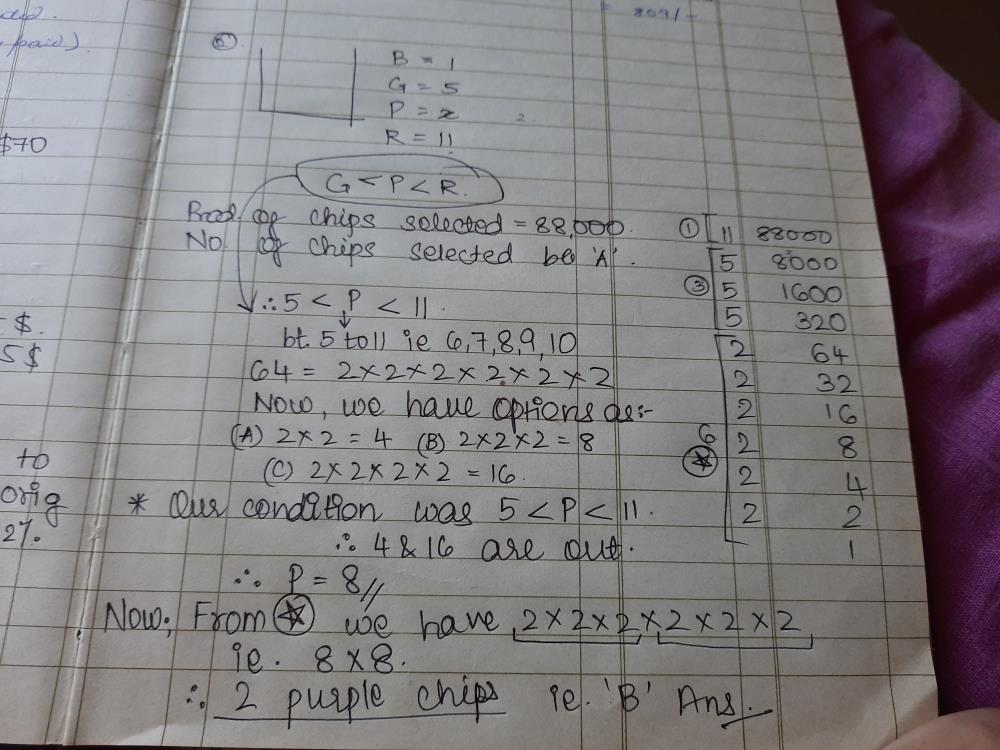Courses

# In a certain game, a large bag is filled with blue, green, purple and red chips worth 1, 5, x and 11 points each, respectively. The purple chips are worth more than the green chips, but less than the red chips. A certain number of chips are then selected from the bag. If the product of the point values of the selected chips is 88,000, how many purple chips were selected? a)1b)2c)3d)4Correct answer is option 'B'. Can you explain this answer? Related Test: Test: Factors And Multiples- 3

## GMAT Question

By Aaditya Sharma · 4 weeks ago ·GMAT
88000=2^6*11*5^3
5< />< />
so x can from 6 to 10

but 88000 has no factor as 3,7
so 6,7,9 are out
rest are 8 and 10
so if I choose x as 10 ,I have 3 quantity of 2 and 5 to be selected that will lead to 8 and 11 multiplication.but we know that no chips is of value 8 and I have already selected x as 10.so x can't be 10.then the last option x as 8 , which can be 2 quantity of 2^3.
so 2 is the right answer.no calculation required.this is a logic based test of math.

Urja Palkar answered 4 weeks ago